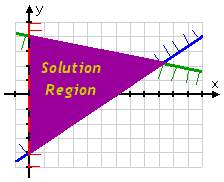Search

Systems of Linear Inequalities (page 1 of 2)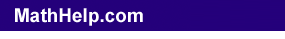Once you've learned how to graph linear inequalities, you can move on to solving systems of linear inequalities.

A "system" of linear inequalities is a set of linear inequalities that you deal with all at once. Usually you start off with two or three linear inequalities. The technique for solving these systems is fairly simple. Here's an example.

• Solve the following system:
• 2x  3y < 12
x + 5y < 20
x > 0

Just as with solving single linear inequalities, it is usually best to solve as many of the inequalities as possible for "y" on one side. Solving the first two inequalities, I get the rearranged system:

y > ( 2/3 )x  4
y < (  1/5 )x + 4

"Solving" systems of linear inequalities means "graphing each individual inequality, and then finding the overlaps of the various solutions". So I graph each inequality, and then find the overlapping portions of the solution regions.

 The line for the first inequality in the above system,  y > ( 2/3 )x  4, looks like this: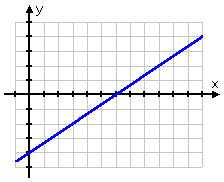This inequality is a "greater than" inequality, so I want to shade above the line. However. since there will be more than one inequality on this graph, I don't know (yet) how much of that upper side I will actually need. Until I know, I can keep track of the fact that I want the top region by drawing a little "fringe" along the top side of the line, like this: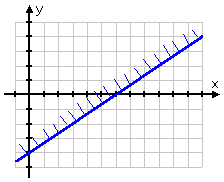Now I graph the line for the second inequality above,  y < (  1/5 )x + 4: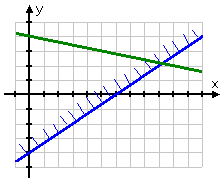...and, since this is a "less than" inequality, I'll draw the fringe along the bottom of the line: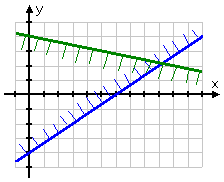The last inequality is a common "real life" constraint: only allowing x to be positive.  The line "x = 0" is just the y-axis, and I want the right-hand side. I need to remember to dash the line in, because this isn't an "or equal to" inequality, so the boundary (the line) isn't included in the solution: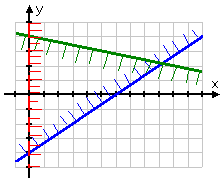The "solution" of the system is the region where all the inequalities are happy; that is, the solution is where all the inequalities work, the region where all three individual solution regions overlap. In this case, the solution is the shaded part in the middle: